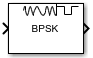# BPSK Demodulator Baseband

Demodulate BPSK-modulated data

• Library:
• Communications Toolbox / Modulation / Digital Baseband Modulation / PM

Communications Toolbox HDL Support / Modulation / PM

•## Description

The BPSK Demodulator Baseband block demodulates a signal that was modulated using the binary phase shift keying method. The input is a baseband representation of the modulated signal. This block accepts a scalar or column vector input signal. The input signal must be a discrete-time complex signal. The block maps the points exp(jθ) or -exp(jθ) to 0 and 1, respectively. The ```Phase offset (rad)``` parameter specifies the value of θ.

## Ports

### Input

expand all

BPSK-modulated signal, specified as a scalar, vector, or matrix. When this input is a matrix, each column is treated as an independent channel. This port is unnamed until the `Var` port is enabled. When the noise variance or signal power result in computations involving extreme positive or negative magnitudes, see BPSK Soft Demodulation for demodulation decision type considerations.

Data Types: `double` | `single` | `fixed point`
Complex Number Support: Yes

Noise variance, specified as a positive scalar or vector of positive values. When the noise variance or signal power result in computations involving extreme positive or negative magnitudes, see BPSK Soft Demodulation for demodulation decision type considerations.

#### Dependencies

To enable this parameter, set the Noise variance source parameter to `Port`.

Data Types: `double`

### Output

expand all

Demodulated signal, returned as a scalar or vector. If the output is a scalar, the value is an integer. If the output is a vector, it is an integer-valued or binary-valued vector.

Data Types: `single` | `double` | `int8` | `int16` | `int32` | `uint8` | `uint16` | `uint32` | `Boolean`

## Parameters

expand all

Main

Decision type used during demodulation, specified as ```Hard decision```, `Log-likelihood ratio` or `Approximate log-likelihood ratio`. For algorithm details, see the Exact LLR Algorithm and Approximate LLR Algorithm topics.

The output matches the data type of the input values when Decision type is set to `Log-likelihood ratio` or `Approximate log-likelihood ratio`.

Noise variance source, specified as `Dialog` or `Port`.

Noise variance, specified as a positive scalar or vector of positive values.

• When specified as a scalar, that value is used on all elements in the input signal.

• When specified as a vector, the vector length must be equal to the number of columns in the input signal. Each noise variance vector element is applied to its corresponding column in the input signal.

When the noise variance or signal power result in computations involving extreme positive or negative magnitudes, see BPSK Soft Demodulation for demodulation decision type considerations.

This parameter is tunable in normal mode, accelerator mode and rapid accelerator mode. If you use the Simulink® Coder™ rapid simulation (RSIM) target to build an RSIM executable, then you can tune the parameter without recompiling the model. This is useful for Monte Carlo simulations, in which you run the simulation multiple times (perhaps on multiple computers) with different amounts of noise.

Tunable: Yes

#### Dependencies

To enable this parameter, set the `Decision type` parameter to set to either `Log-likelihood ratio` or `Approximate log-likelihood ratio` and set the Noise variance source parameter to `Dialog`.

Phase of the zeroth point, specified as a real-valued scalar. Units are in radians.

Example: `pi/4`

Data Types

Output data type, specified as one of these options

• When you set the Decision type parameter to Hard decision:

• `Inherit via internal rule` — The block inherits the output data type from the input port. If the input is a floating-point type (`single` or `double`), the output data type is the same as the input data type. If the input data type is fixed-point, the output data type works as if you set this parameter to ```Smallest unsigned integer```.

• `Smallest unsigned integer` — The block selects the output data type based on the settings used in the Hardware Implementation pane of the Configuration Parameters dialog box of the model. If you select `ASIC/FPGA` in the Hardware Implementation pane, the output data type is the ideal minimum one-bit size, that is, `ufix(1)`. For all other selections, the output data type is an unsigned integer with the smallest available word length large enough to fit one bit, usually corresponding to the size of a character (for example, `uint8`).

• `double`

• `single`

• `int8`

• `uint8`

• `int16`

• `uint16`

• `int32`

• `uint32`

• `boolean`

• When you set the Decision type parameter to Log-likelihood ratio or Approximate log-likelihood ratio — The block inherits the output data type matches the data type of the input.

Derotate factor, specified as ```Same word length as input``` or ```Specify word length```.

#### Dependencies

This parameter applies only when the input is fixed-point and the Phase offset (rad) parameter is not a multiple of π/2.

## Block Characteristics

 Data Types `Boolean` | `double` | `fixed point[a][b]` | `integer` | `single` Multidimensional Signals `no` Variable-Size Signals `yes` [a] Fixed-point inputs must be signed.[b] ufix(1) only at the output when ASIC/FPGA is selected in the Hardware Implementation Pane.

expand all

## Extended Capabilities

### Topics

Introduced before R2006a